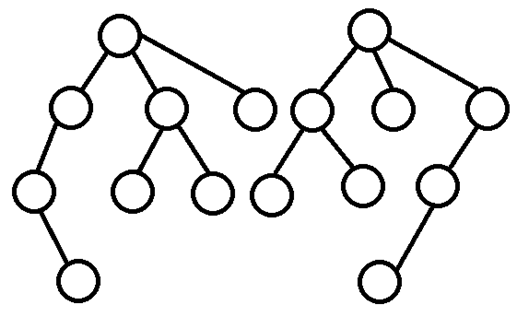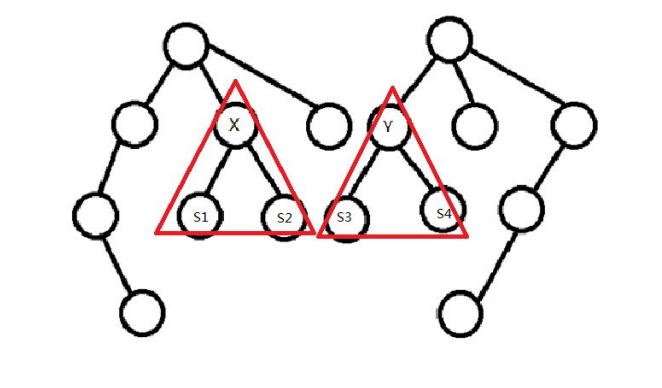# [JZOJ4272] [NOIP2015模拟10.28B组] 序章-弗兰德的秘密 解题报告(树形DP)

#### Description

1、两棵树节点个数相等。
2、两棵树的以根节点的儿子为根子树对应同构。如下图，为两棵同构的有根树。#### Sample Input

3 31 21 31 22 3

#### Sample Output

2【样例解释】第一棵树可以保留1号节点和2号节点删除3号节点，也可以保留1号节点与3号节点删除2号节点，第二棵树保留1号节点和2号节点删除3号节点。剩下的树同构，树的节点个数均为2。

#### Data Constraint30%:直接上暴力。枚举两颗树中保留哪些节点，求最大的同构。

100%：树型 DP。设状态F[x][y]表示，第一棵树中x节点与第二棵树中的y节点作为根节点匹配的最大同构。例如，上图中所示的F[x][y] = 3。现在转移方程就很显然了： F[x][y]=Max{F[x的儿子][y的儿子]}+1 ; 这里需要枚举x的那些儿子与y的哪些儿子匹配，这部分时间复杂度O(5!) ; 最后答案就是F. 总的时间复杂度为O(5!*n2

#include<iostream>
#include<cstdio>
#include<algorithm>
#include<cstring>
#include<vector>
using namespace std;

const int maxn=1000+15;
int n,m,x,y;
int f[maxn][maxn],vis[maxn];
vector <int> son1[maxn];
vector <int> son2[maxn];
{
char ch=getchar();
int s=0,f=1;
while (!(ch>='0'&&ch<='9')) {if (ch=='-') f=-1;ch=getchar();}
while (ch>='0'&&ch<='9') {s=(s<<3)+(s<<1)+ch-'0';ch=getchar();}
return s*f;
}
void dfs1(int k,int s)
{
if (k>son1[x].size()) {f[x][y]=max(f[x][y],s);return;}//当点x的孩子访问完时，是时候返回值了
dfs1(k+1,s);
bool flag=true;
for (int j=0;j<son2[y].size();j++)
if (!vis[j])
{
vis[j]=1;
flag=false;
dfs1(k+1,s+f[son1[x][k-1]][son2[y][j]]);//加上子节点的最大同构值
vis[j]=0;
}
if (flag) f[x][y]=max(f[x][y],s);
}
void dfs(int k)
{
if (!son1[k].size())//当k节点没有孩子时
{
for (int i=1;i<=m;i++)
f[k][i]=1;//只有k节点一个可以同构
return;
}
for (int i=0;i<son1[k].size();i++)
dfs(son1[k][i]);
for (int i=1;i<=m;i++)
{
if (!son2[i].size()) f[k][i]=1;
else
{
x=k;y=i;
dfs1(1,0);
f[k][i]++;//最后算上k节点一个
}
}
}
int main()
{
//    freopen("frand.in","r",stdin);
//    freopen("frand.out","w",stdout);
for (int i=1;i<n;i++)
{
son1[xx].push_back(yy);
}
for (int i=1;i<m;i++)
{
}